# Timeseries Stationarity

This blog post discusses the importance of stationarity in timeseries data when performing analysis or generating forecasts. Non-stationary data can pose challenges for accurate forecasting, but transformations such as differencing, Box-Cox, and Yeo-Johnson can help make data stationary. These techniques can help with effective planning and implementation of energy management policies.Timeseries (a sequence of datapoints ordered in time) analysis and forecasting techniques are commonly used to analyse and predict energy consumption, demand, and production. Timeseries analysis involves studying the patterns, trends, and behaviour of these data points over time to identify and forecast future values.

This blog post will be looking at the importance of stationarity in timeseries data when performing analysis or generating forecasts.

## Stationarity

Timeseries data can often be non-stationary, which can pose challenges for accurate forecasting. Stationarity refers to the property of a timeseries where its statistical properties, such as mean and variance, do not change over time. This makes it easier for predictive models to identify trends and patterns.

We can apply transformations to scale the data points, approximating them to a normal distribution, making data points stationary.

## Differencing

The simplest transformation we can apply to a timeseries dataset is differencing. To difference a time series, we take the difference between consecutive observations. This has the effect of removing the trend or seasonality from the data and making the series stationary. If the first order difference does not produce a stationary series, we can try differencing the series again until we achieve stationarity.

Below is the function applied to all data points, where y’ is the differenced value, and yi is the original value at time i. NumPy and Pandas both have functions that automate this for ease of implementation.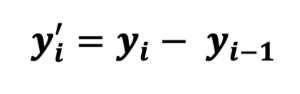## Box Cox Transformation

The Box-Cox transformation is a popular power transform, that can effectively make data stationary, and approximate it to the normal distribution.

Below is the function applied to all data points, where y is the original data, and lambda is the transformation parameter. Lambda varies between -5 to 5, and through Maximum Likelihood Estimation, the best resulting normal distribution approximation for the given value of lambda is chosen. SciPy and Scikit Learn both have functions that automate this.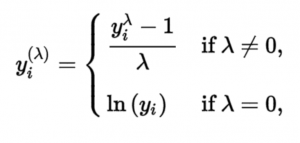## Yeo-Johnson Transformation

Yeo-Johnson is another popular power transform and is very similar to the Box-Cox transformation. Yeo-Johnson extends on Box-Cox, by adding support for negative values. For positive and zero values it follows the same logic, but has an additional, different function for negative values.

Again, the image below shows the function applied to all data points, where y is the original data, and lambda is the transformation parameter. As before, lambda varies between -5 to 5, and through Maximum Likelihood Estimation, the best resulting normal distribution approximation for the given value of lambda is chosen. SciPy and Scikit Learn both have functions that automate this.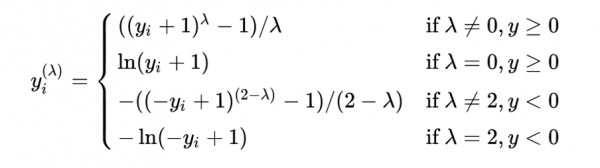## Augmented Dickey-Fuller Test

After we have applied a transformation, it is vital to assess how stationarity the result is. We can do this with an Augmented Dickey-Fuller Test.

The ADF Test is a regression-based test that compares the lagged differences of the time series against the null hypothesis of a unit root. Specifically, the test fits a regression model to the time series data and tests whether the coefficient on the lagged difference term is significantly different from zero. If the coefficient is significantly different from zero, it suggests that the time series is stationary and does not have a unit root. Statsmodels has a function that automates this.

## Inversing Transformations

When using any of these transformations for analytics or predictions, they can be inversed afterwards. This gives more interpretable results, that are of the same magnitude as your original data.

## Example

Below is a worked example of assessing stationarity and applying transformations in Python. The dataset used is sourced from here, under CC0 licensing. You can also see the whole notebook on GitHub here.

```				```
import pandas as pd
from matplotlib import pyplot as plt
plt.rcParams["figure.figsize"] = (10, 5)

df.columns = ["Date", "Count"]
df.set_index("Date", inplace=True)

# Plot
```
```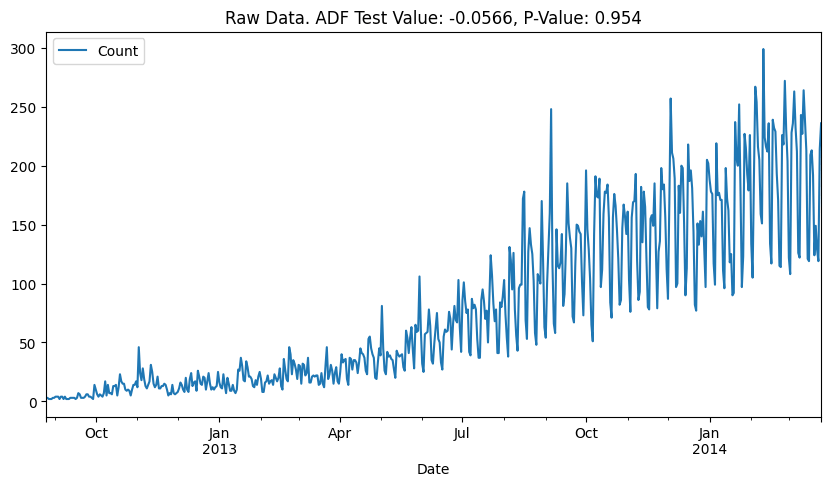Here we can see the raw data. We can define the following hypotheses:

H0: The variance and mean are not constant, and the timeseries is not stationary.

H1: The variance and mean are constant, and the timeseries is stationary.

Looking at the graph, the variance and mean are clearly not constant. We can confirm that we fail to reject the null hypothesis at a 1% significance level using the Augmented Dickey-Fuller Test’s P-Value, as 0.954 > 0.01.

Let’s apply some of the transformations we have learnt about and see if we can improve this!

```				```
from scipy.stats import boxcox

# Apply Box-Cox transformation
df["Count_BoxCox"], _ = boxcox(df["Count"])

# Plot
```
```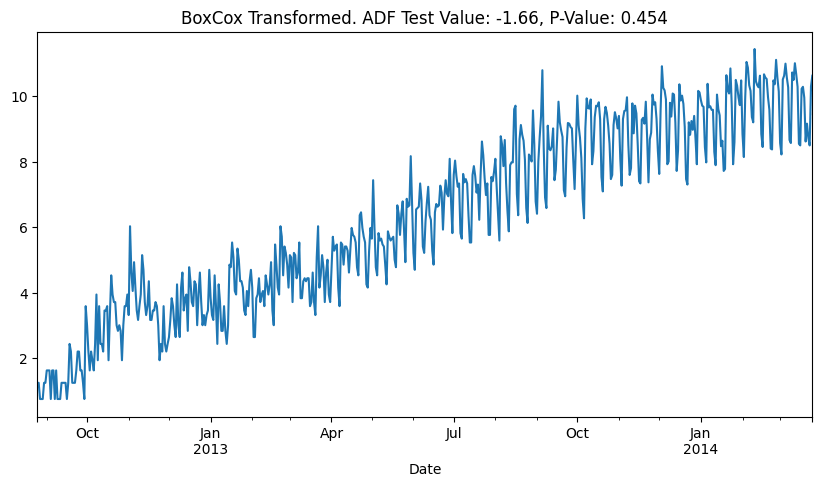Looking at the graph, the Box-Cox transformation has clearly adjusted the variance of the data. It now looks a lot more constant than before. However, our mean still varies throughout time.

The ADF Test’s P-Value confirms this, as we fail to reject the null hypothesis, as 0.454 > 0.01.

Let’s try another transformation, to see if we can improve further.

```				```
# Apply differencing
df["Count_BoxCox_Diff"] = df["Count_BoxCox"].diff()

# Plot results
```
```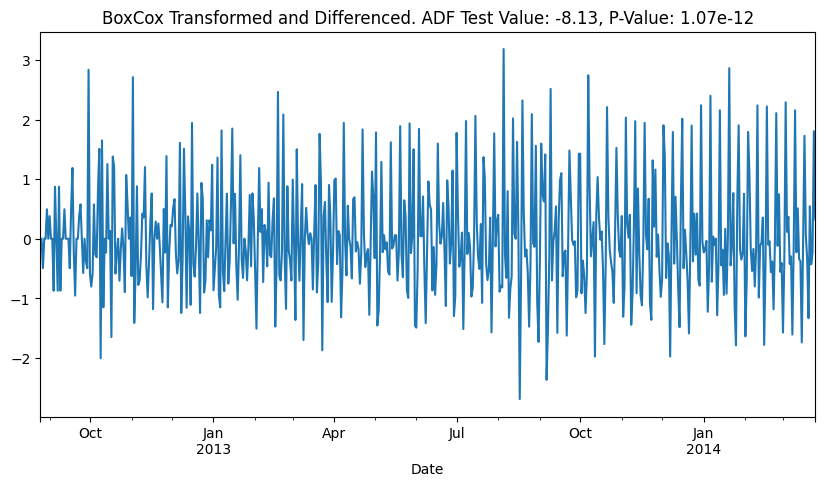This looks a lot better, now the variance and mean appear constant from the graph.

The ADF Test’s P-Value confirms this, as 1.07e-12 < 0.01, we can now reject the null hypothesis, at a 1% significance level.

This data is now ready for analysis and predictions, as the time component has been factored out.

## Conclusion

In conclusion, transformations such as differencing, the Box-Cox and Yeo-Johnson transformations are useful tools in generating accurate forecasts for timeseries data. By transforming non-stationary data into stationary data with a normal distribution, it becomes easier for predictive models to identify trends and patterns. The Augmented Dickey-Fuller test is a useful tool to verify the success of these transformations. With the availability of built-in functions in popular Python libraries such as SciPy and Scikit Learn, implementing these transformations has become easy and accessible to data analysts and researchers alike.

https://otexts.com/fpp2/stationarity.html

https://machinelearningmastery.com/time-series-data-stationary-python/Angus Doherty